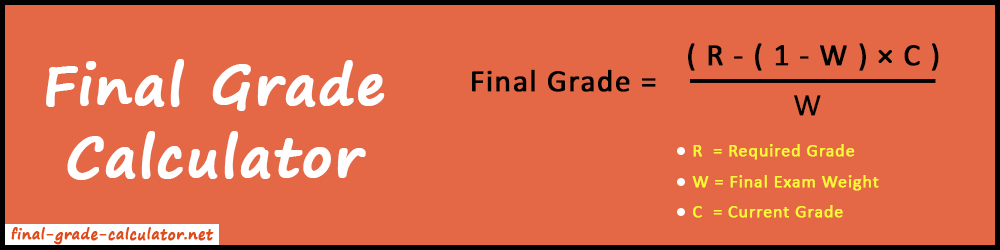Final Grade Calculator helps you to find what grade you need on your final exam to achieve the desired score.

%
%
%
%
%
%
%
%
 On Current Grade (letter) Current Grade (%) Weight Desired Grade (letter) Desired Grade (%) Total Weights -- A+ A A- B+ B B- C+ C C- D+ D D- F Final Exam Grade Final Exam Grade Final Exam Weight
##### Related Tools
Grade Calculator High School GPA Calculator

Final grade calculator will take your final exam preparation to new heights. You can use this online tool to find the grade score needed to achieve your desired grade in the final exams.

In short, it will tell you the difference between your current grade and desired grade according to the total weightage. In this way, you can more specifically set your targets and work on them. Also, our tool uses a simple formula to evaluate the final exam grade using the values of required grade, current grade, and final exam weight.### How to Calculate Final Grade?

Firstly, Let's have a closer look at the formula to determine the final grade.

#### Formula:

F =
 ( R - ( 1 - W ) × C ) W

Where,

R = Required Grade that you want for a class
W = Final Exam Weight

However, it seems to be a simple calculation and but it's time-consuming. Whereas the Final Grade Calculator will give you the same answer in less than a second.

Let's understand the working of the formula with example.

##### Example:

Jerry studies in school, his current score in Physics is 77%. He is dissatisfied with his current score and aims to score at least 85%. The final exam weightage in his school is 45%. Find out the grade (%) Jerry needs to score to achieve his target.

##### Solution:

Here,
Final Exam Weight (W) = 45% = (Convert into decimal: 45 / 100 = 0.45)

Note: Make sure that the final exam weight(W) must be in decimal form. For that, you can divide the percent value by 100 and convert it into a decimal.

Now, apply all the values to the formula to find the final exam grade.

F =
 (R - (1 - W) × C) W
F =
 (85 - (1 - 0.45) × 77) 0.45
F =
 (85 - (0.55 × 77)) 0.45
F =
 (85 - 42.35) 0.45
F =
 42.65 0.45

So, Jerry requires to score 94.78% in the final exam to achieve his target of 85%.

As you can see above, the mathematical calculation can be time taking and a little bit confusing. Hence, we built the easy-to-use final grade calculator for your convenience. Keep it handy or bookmark it for future use.

### How to Use the Final Grade Calculator?

In the final grade calculator, there are three tabs for three types of calculations.

1. FinalEach tab does different tasks and uses different formulas. The first tab is the Final tab which is the default tab. If you want to simply find the final grade then you can go with it. However, for even more you can go for Overall Grade or Multiple Grade tabs. Let's know about each of them one by one.

#### 1. Final

It is used to find the grade needed in the final exam to achieve the desired grade. This tab has three input boxes: Current Grade, Desired Grade, and Final Exam Weight. Fill these three input boxes with the correct value and click on the "Calculate" button to get the final grade needed.This calculator is used to find the overall course grade when you have already given the final exam. It also has three input boxes: Current Average Grade, Final Exam Grade, and Final Exam Weight. Enter values in these three boxes and press the "Calculate" button to get the overall course grade.